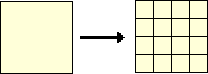Definition Of Area

Area is defined as the number of square units that covers a closed figure.

Examples of Area

In the example shown, the area of the yellow square is 16 square units. That means, 16 square units are needed to cover the surface enclosed by the square.Formulas are defined to calculate the area of regular geometric figures like square, rectangle, circle, etc.

Solved Example Clockple on Approximate

Ques: Find the area of a rectangle 13 cm long and 9 cm wide.

Choices:

A. 97 square cm
B. 22 square cm
C. 44 square cm
D. 117 square cm